Скачать презентацию Banking in the US All Banks in

eeb07a95e7de8088678f459f4798f922.ppt

• Количество слайдов: 56Banking in the US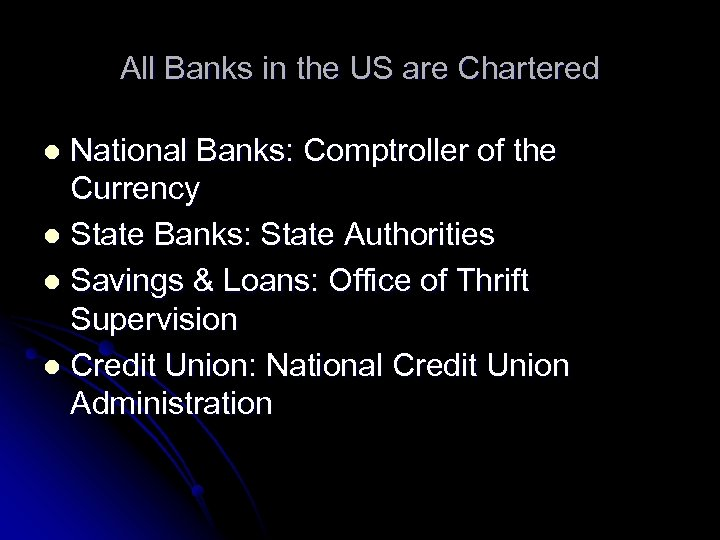All Banks in the US are Chartered National Banks: Comptroller of the Currency l State Banks: State Authorities l Savings & Loans: Office of Thrift Supervision l Credit Union: National Credit Union Administration l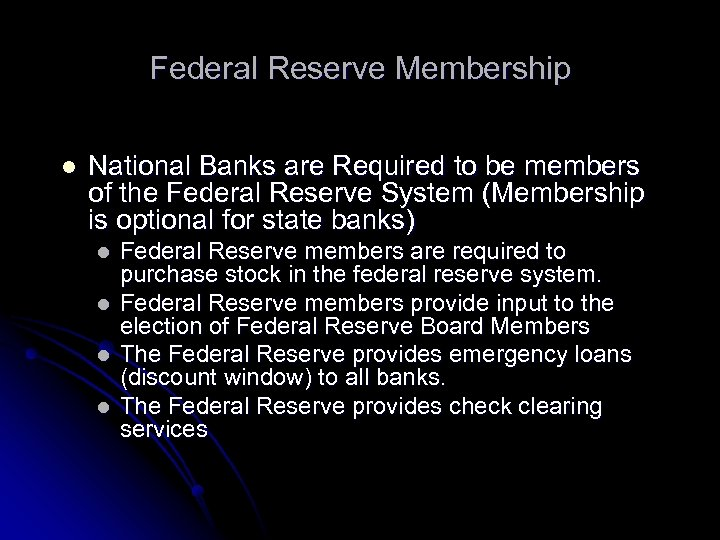Federal Reserve Membership l National Banks are Required to be members of the Federal Reserve System (Membership is optional for state banks) l l Federal Reserve members are required to purchase stock in the federal reserve system. Federal Reserve members provide input to the election of Federal Reserve Board Members The Federal Reserve provides emergency loans (discount window) to all banks. The Federal Reserve provides check clearing services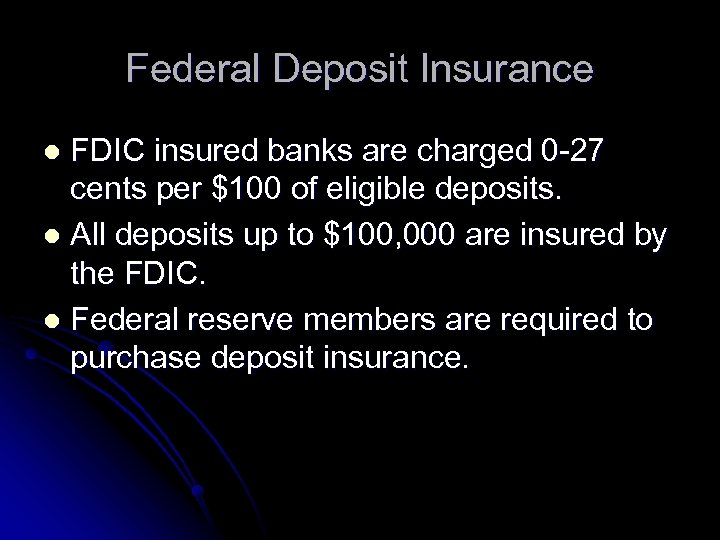Federal Deposit Insurance FDIC insured banks are charged 0 -27 cents per \$100 of eligible deposits. l All deposits up to \$100, 000 are insured by the FDIC. l Federal reserve members are required to purchase deposit insurance. l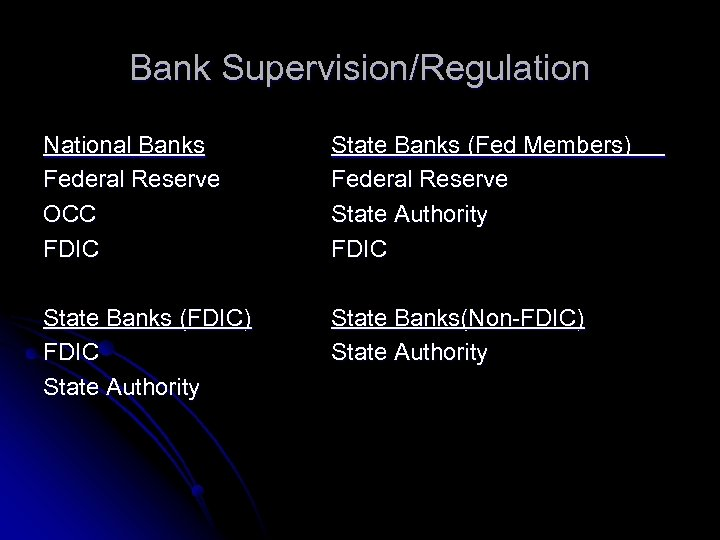Bank Supervision/Regulation National Banks Federal Reserve OCC FDIC State Banks (Fed Members) Federal Reserve State Authority FDIC State Banks (FDIC) FDIC State Authority State Banks(Non-FDIC) State Authority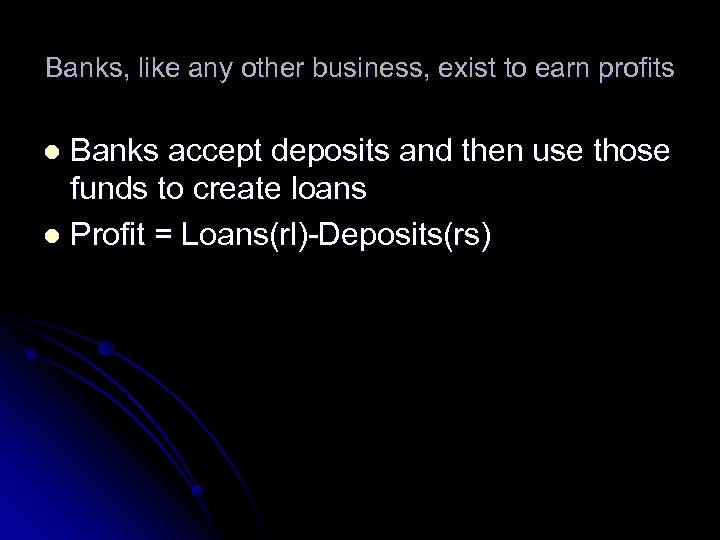Banks, like any other business, exist to earn profits Banks accept deposits and then use those funds to create loans l Profit = Loans(rl)-Deposits(rs) l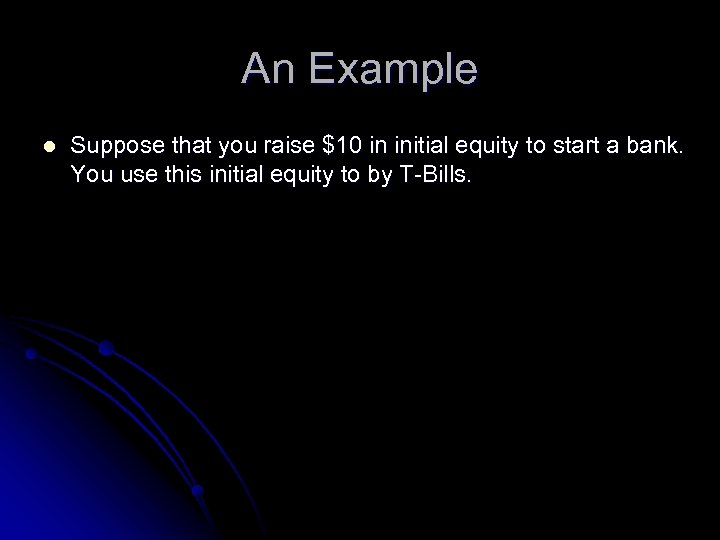An Example l Suppose that you raise \$10 in initial equity to start a bank. You use this initial equity to by T-Bills.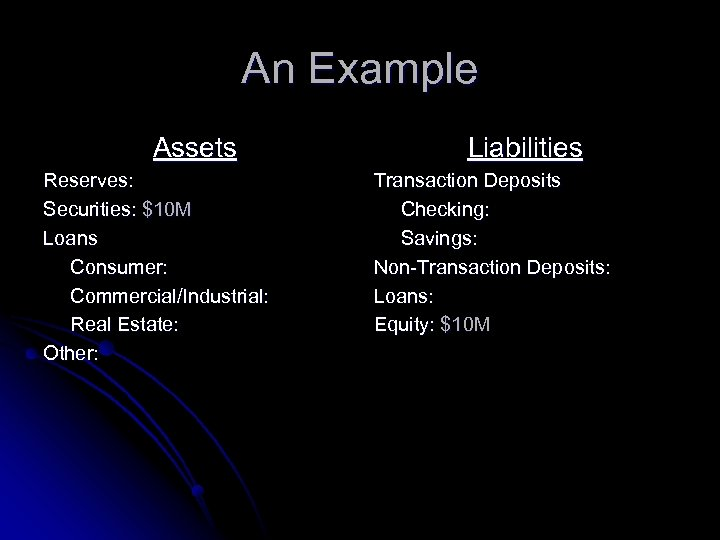An Example Assets Reserves: Securities: \$10 M Loans Consumer: Commercial/Industrial: Real Estate: Other: Liabilities Transaction Deposits Checking: Savings: Non-Transaction Deposits: Loans: Equity: \$10 M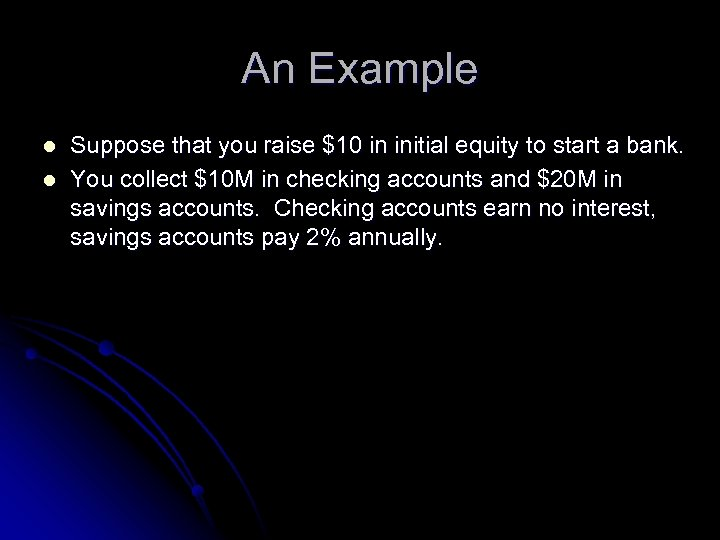An Example l l Suppose that you raise \$10 in initial equity to start a bank. You collect \$10 M in checking accounts and \$20 M in savings accounts. Checking accounts earn no interest, savings accounts pay 2% annually.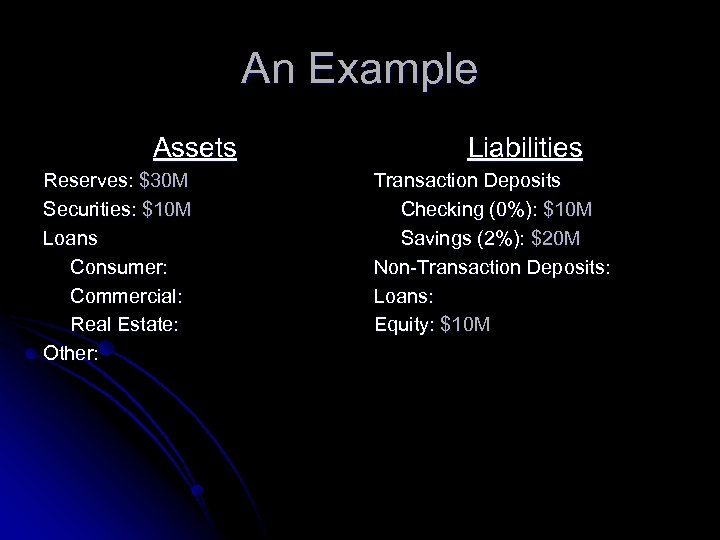An Example Assets Reserves: \$30 M Securities: \$10 M Loans Consumer: Commercial: Real Estate: Other: Liabilities Transaction Deposits Checking (0%): \$10 M Savings (2%): \$20 M Non-Transaction Deposits: Loans: Equity: \$10 M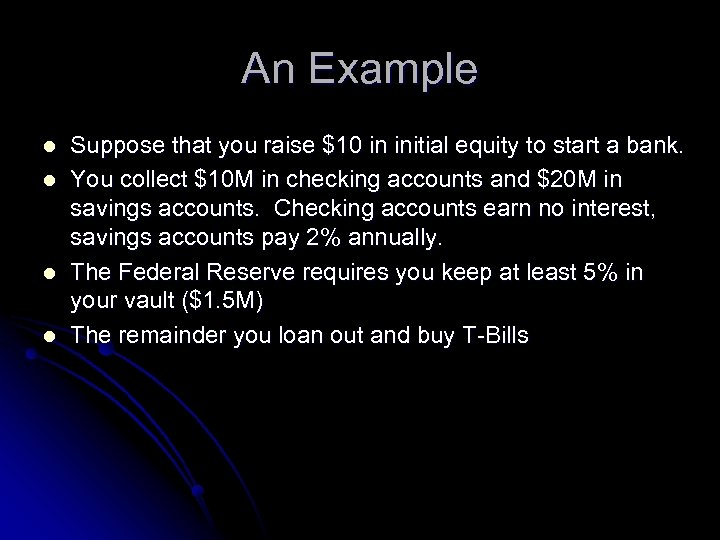An Example l l Suppose that you raise \$10 in initial equity to start a bank. You collect \$10 M in checking accounts and \$20 M in savings accounts. Checking accounts earn no interest, savings accounts pay 2% annually. The Federal Reserve requires you keep at least 5% in your vault (\$1. 5 M) The remainder you loan out and buy T-Bills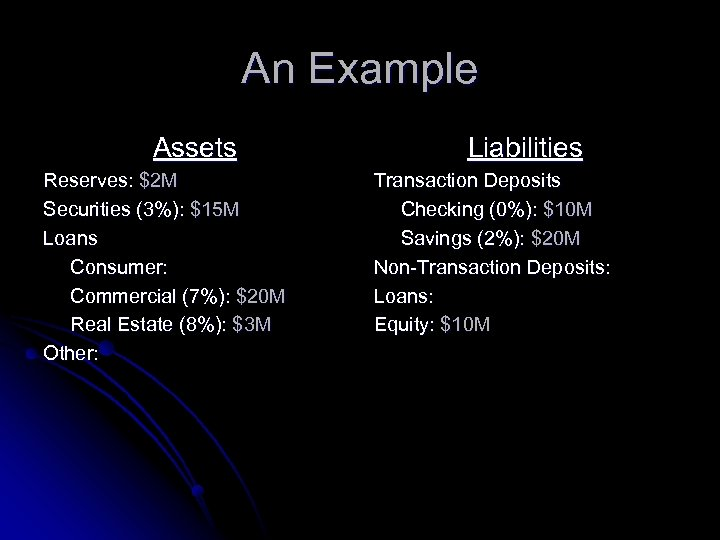An Example Assets Reserves: \$2 M Securities (3%): \$15 M Loans Consumer: Commercial (7%): \$20 M Real Estate (8%): \$3 M Other: Liabilities Transaction Deposits Checking (0%): \$10 M Savings (2%): \$20 M Non-Transaction Deposits: Loans: Equity: \$10 M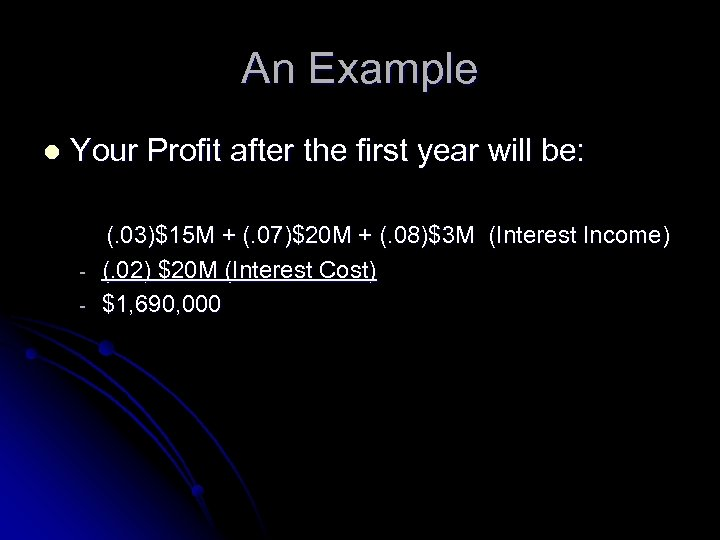An Example l Your Profit after the first year will be: - (. 03)\$15 M + (. 07)\$20 M + (. 08)\$3 M (Interest Income) (. 02) \$20 M (Interest Cost) \$1, 690, 000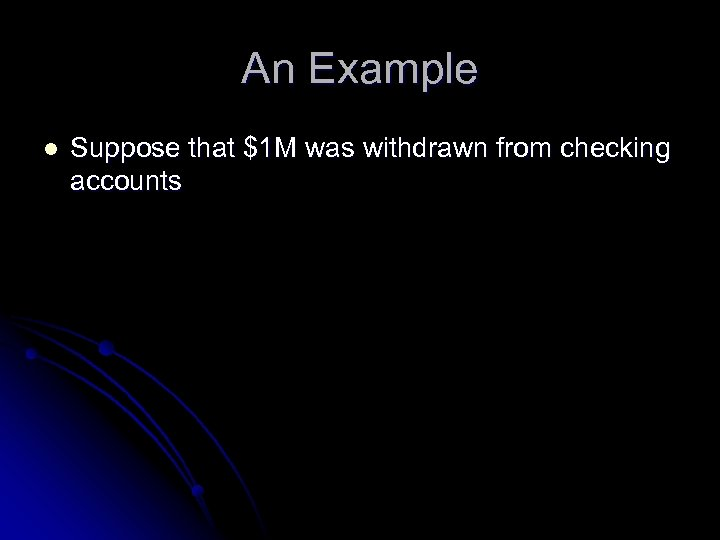An Example l Suppose that \$1 M was withdrawn from checking accounts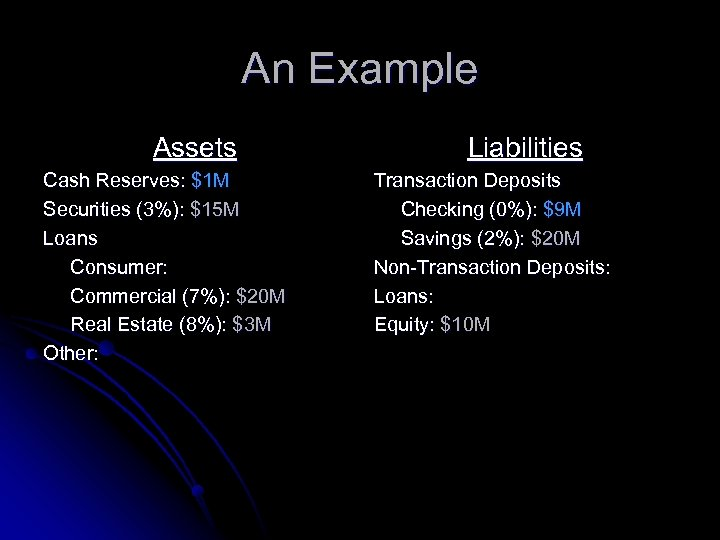An Example Assets Cash Reserves: \$1 M Securities (3%): \$15 M Loans Consumer: Commercial (7%): \$20 M Real Estate (8%): \$3 M Other: Liabilities Transaction Deposits Checking (0%): \$9 M Savings (2%): \$20 M Non-Transaction Deposits: Loans: Equity: \$10 M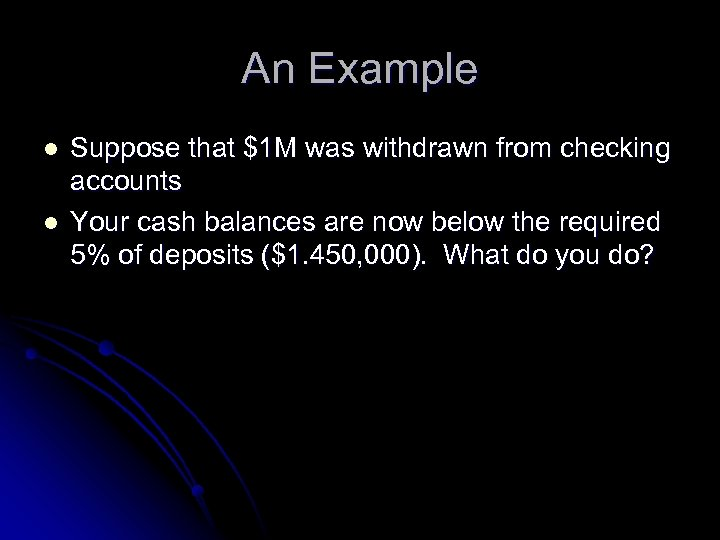An Example l l Suppose that \$1 M was withdrawn from checking accounts Your cash balances are now below the required 5% of deposits (\$1. 450, 000). What do you do?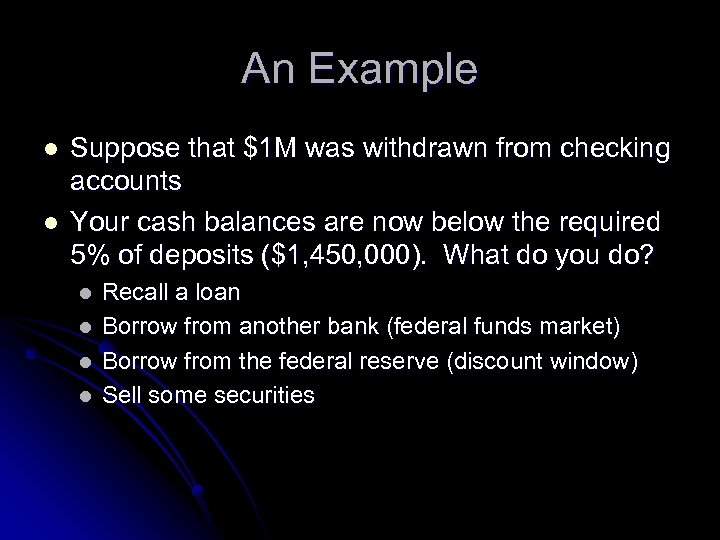An Example l l Suppose that \$1 M was withdrawn from checking accounts Your cash balances are now below the required 5% of deposits (\$1, 450, 000). What do you do? l l Recall a loan Borrow from another bank (federal funds market) Borrow from the federal reserve (discount window) Sell some securities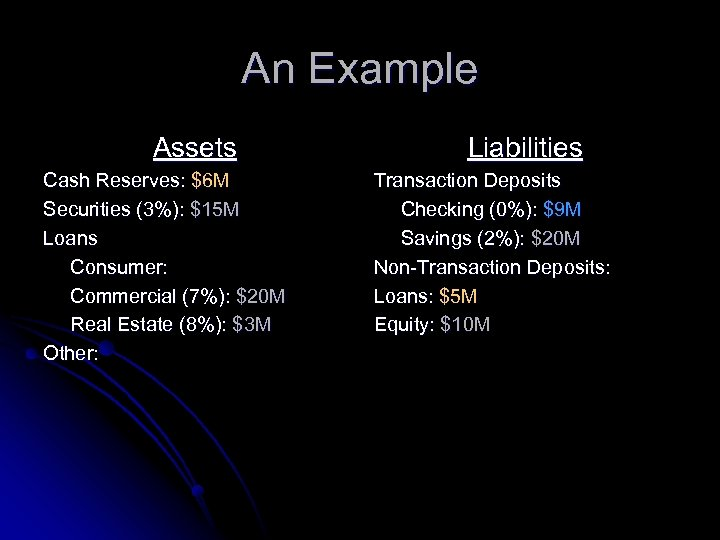An Example Assets Cash Reserves: \$6 M Securities (3%): \$15 M Loans Consumer: Commercial (7%): \$20 M Real Estate (8%): \$3 M Other: Liabilities Transaction Deposits Checking (0%): \$9 M Savings (2%): \$20 M Non-Transaction Deposits: Loans: \$5 M Equity: \$10 M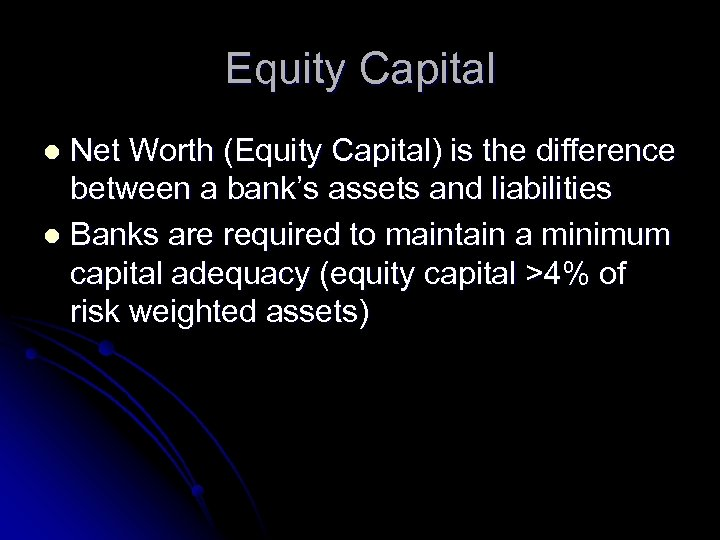Equity Capital Net Worth (Equity Capital) is the difference between a bank’s assets and liabilities l Banks are required to maintain a minimum capital adequacy (equity capital >4% of risk weighted assets) l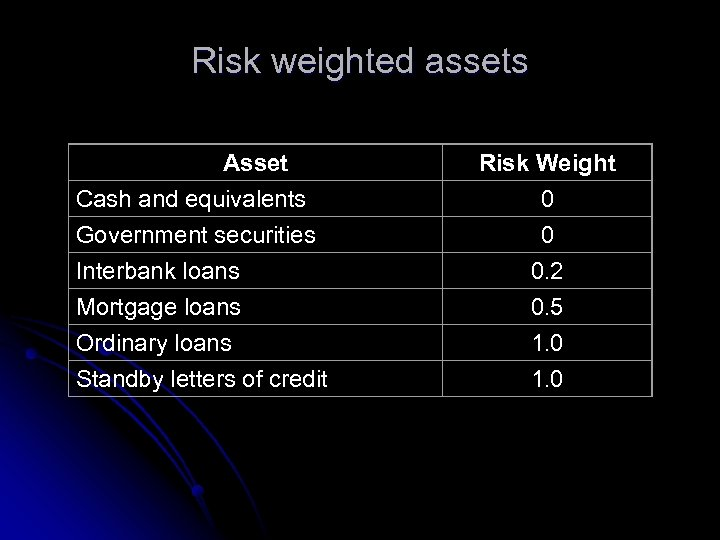Risk weighted assets Asset Cash and equivalents Government securities Interbank loans Mortgage loans Ordinary loans Standby letters of credit Risk Weight 0 0 0. 2 0. 5 1. 0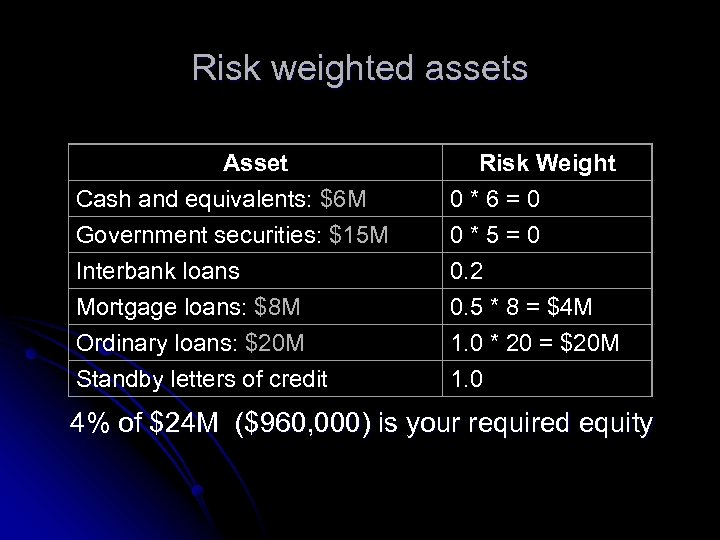Risk weighted assets Asset Cash and equivalents: \$6 M Government securities: \$15 M Interbank loans Mortgage loans: \$8 M Ordinary loans: \$20 M Standby letters of credit Risk Weight 0*6=0 0*5=0 0. 2 0. 5 * 8 = \$4 M 1. 0 * 20 = \$20 M 1. 0 4% of \$24 M (\$960, 000) is your required equity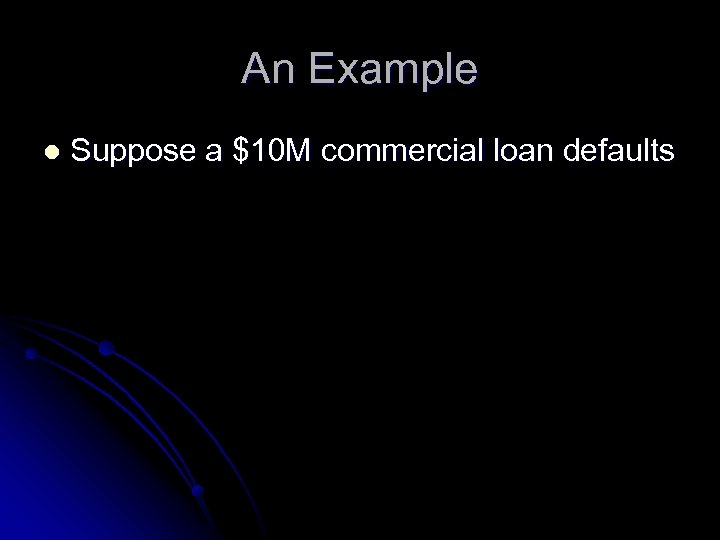An Example l Suppose a \$10 M commercial loan defaults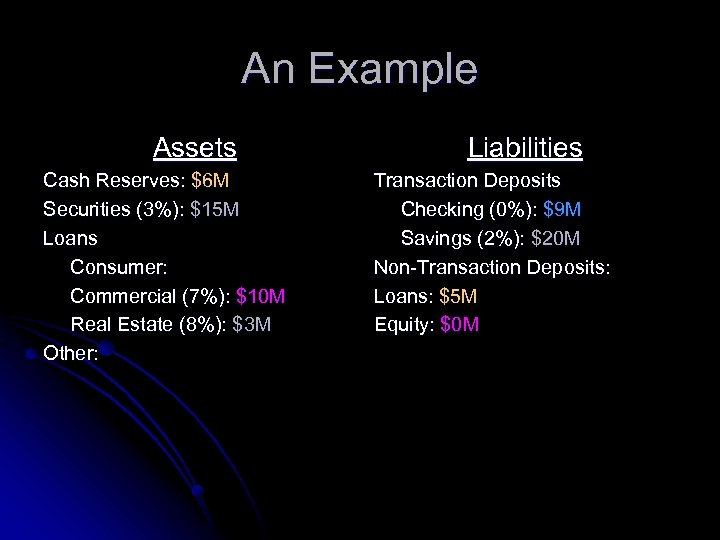An Example Assets Cash Reserves: \$6 M Securities (3%): \$15 M Loans Consumer: Commercial (7%): \$10 M Real Estate (8%): \$3 M Other: Liabilities Transaction Deposits Checking (0%): \$9 M Savings (2%): \$20 M Non-Transaction Deposits: Loans: \$5 M Equity: \$0 M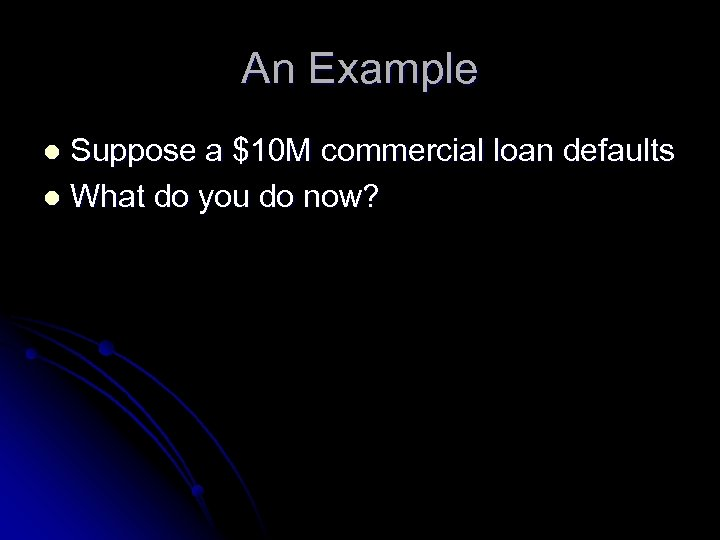An Example Suppose a \$10 M commercial loan defaults l What do you do now? l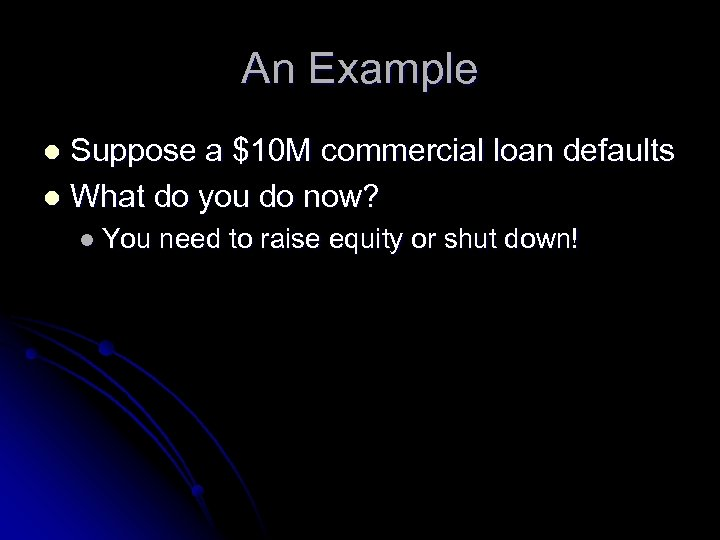An Example Suppose a \$10 M commercial loan defaults l What do you do now? l l You need to raise equity or shut down!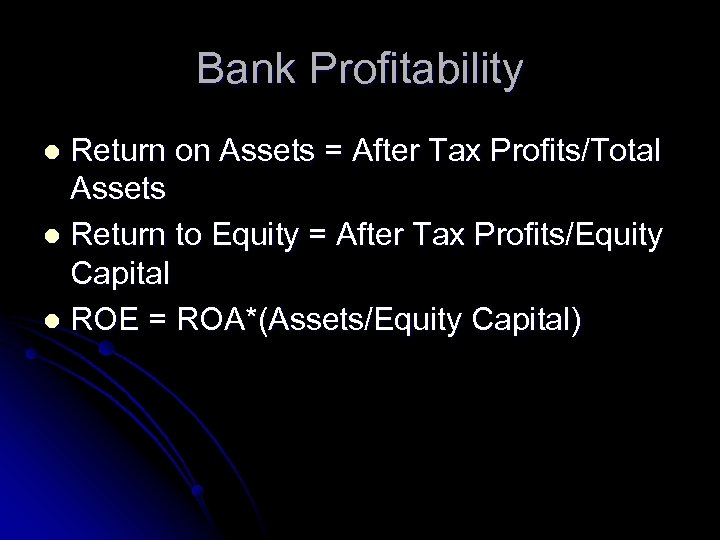Bank Profitability Return on Assets = After Tax Profits/Total Assets l Return to Equity = After Tax Profits/Equity Capital l ROE = ROA*(Assets/Equity Capital) l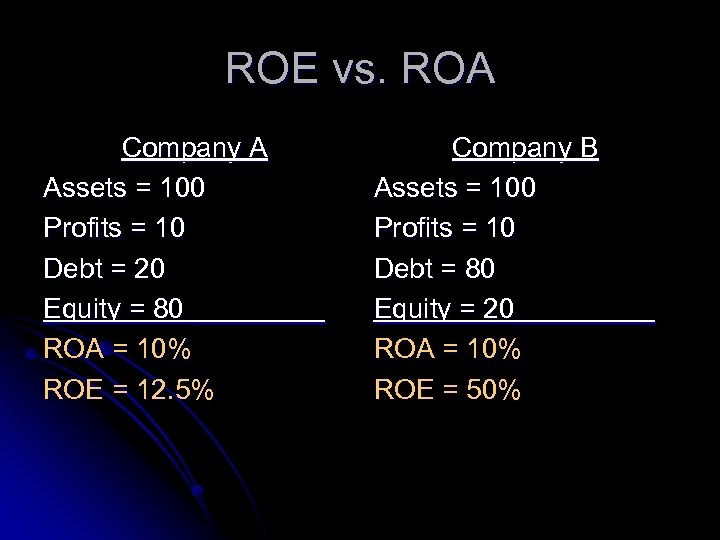ROE vs. ROA Company A Assets = 100 Profits = 10 Debt = 20 Equity = 80_____ ROA = 10% ROE = 12. 5% Company B Assets = 100 Profits = 10 Debt = 80 Equity = 20_____ ROA = 10% ROE = 50%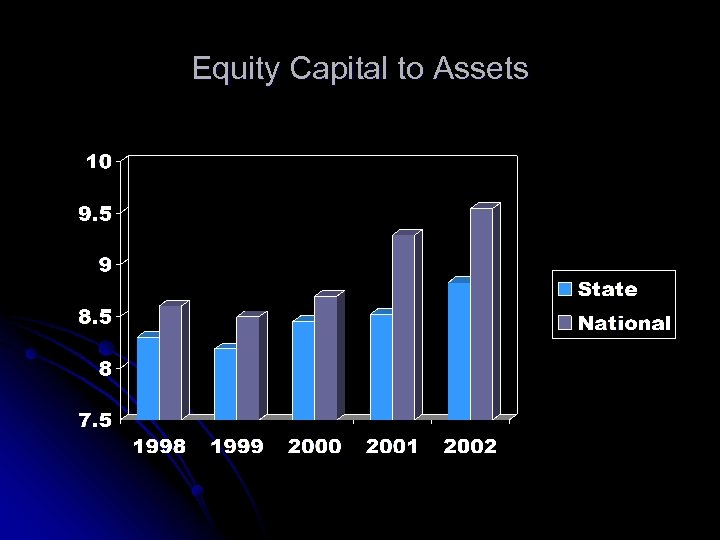Equity Capital to Assets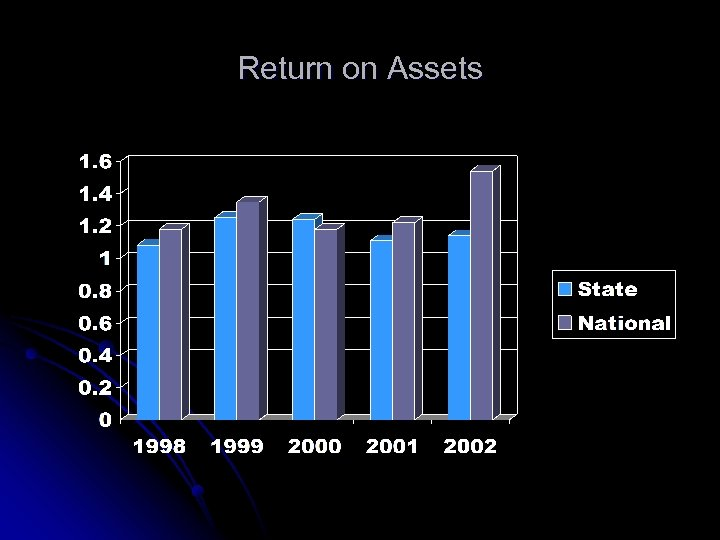Return on Assets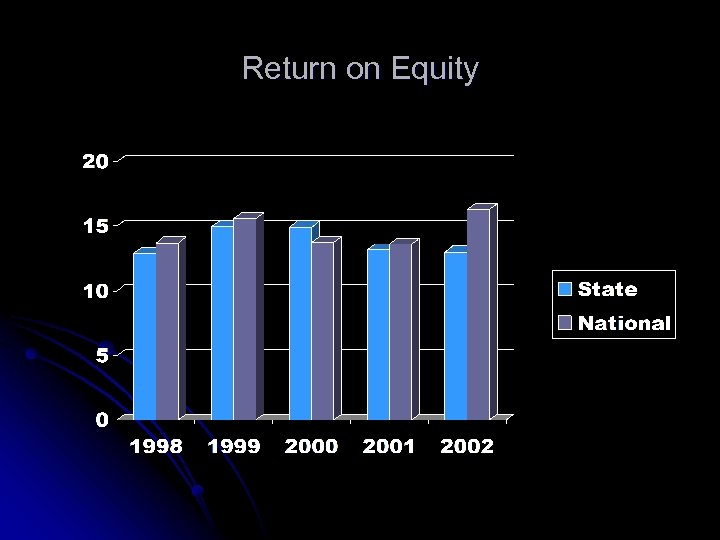Return on Equity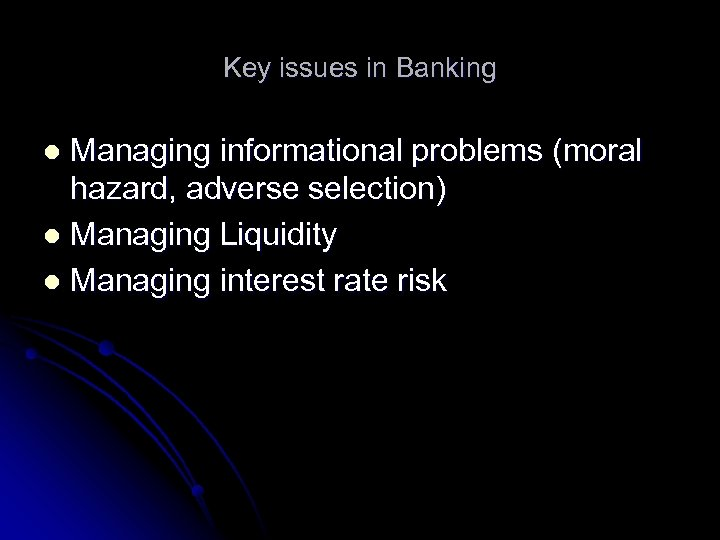Key issues in Banking Managing informational problems (moral hazard, adverse selection) l Managing Liquidity l Managing interest rate risk l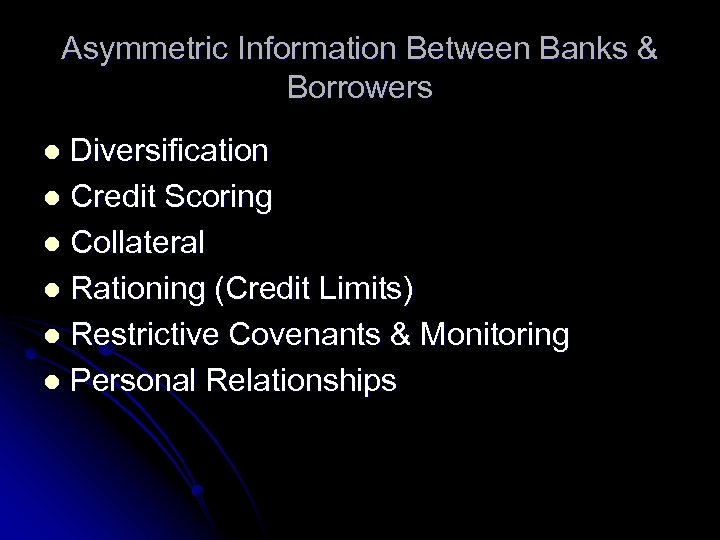Asymmetric Information Between Banks & Borrowers Diversification l Credit Scoring l Collateral l Rationing (Credit Limits) l Restrictive Covenants & Monitoring l Personal Relationships l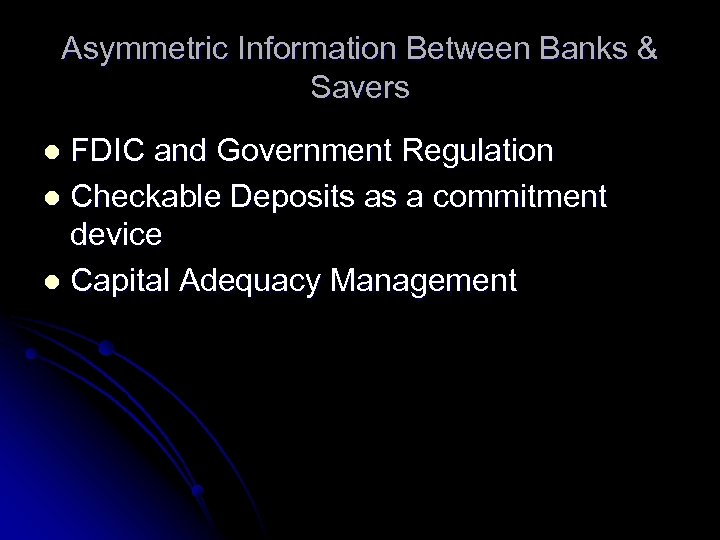Asymmetric Information Between Banks & Savers FDIC and Government Regulation l Checkable Deposits as a commitment device l Capital Adequacy Management l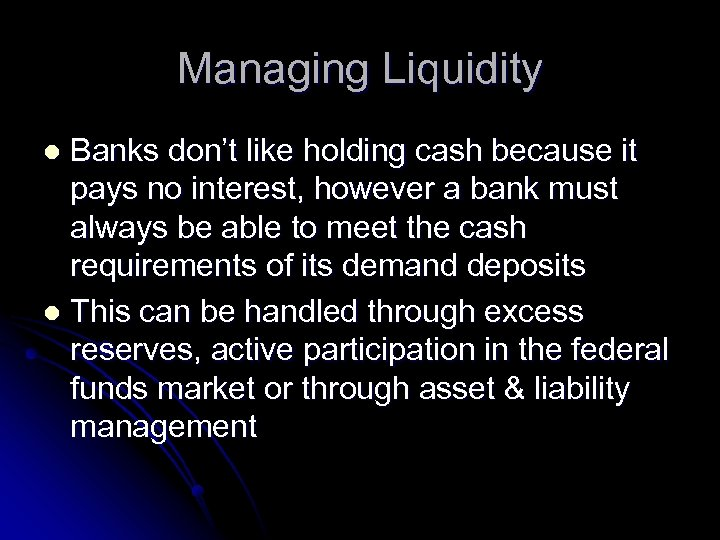Managing Liquidity Banks don’t like holding cash because it pays no interest, however a bank must always be able to meet the cash requirements of its demand deposits l This can be handled through excess reserves, active participation in the federal funds market or through asset & liability management l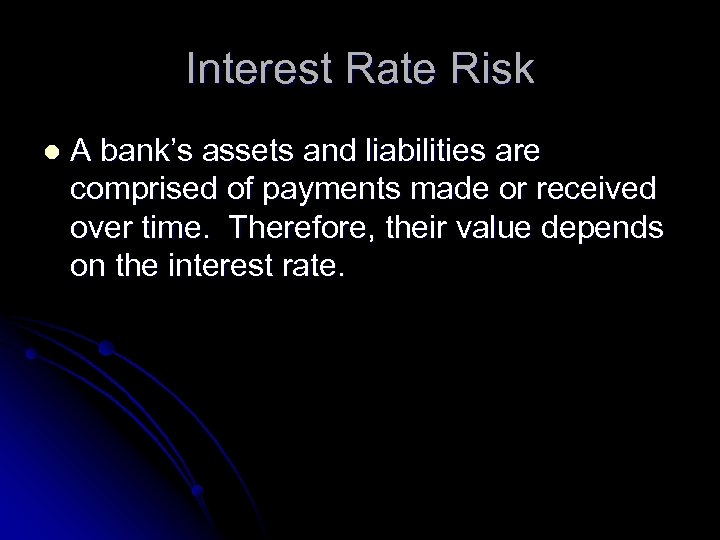Interest Rate Risk l A bank’s assets and liabilities are comprised of payments made or received over time. Therefore, their value depends on the interest rate.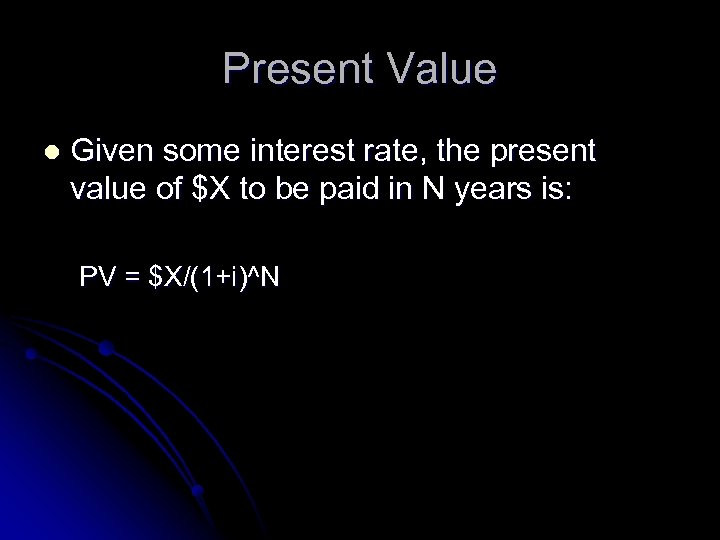Present Value l Given some interest rate, the present value of \$X to be paid in N years is: PV = \$X/(1+i)^N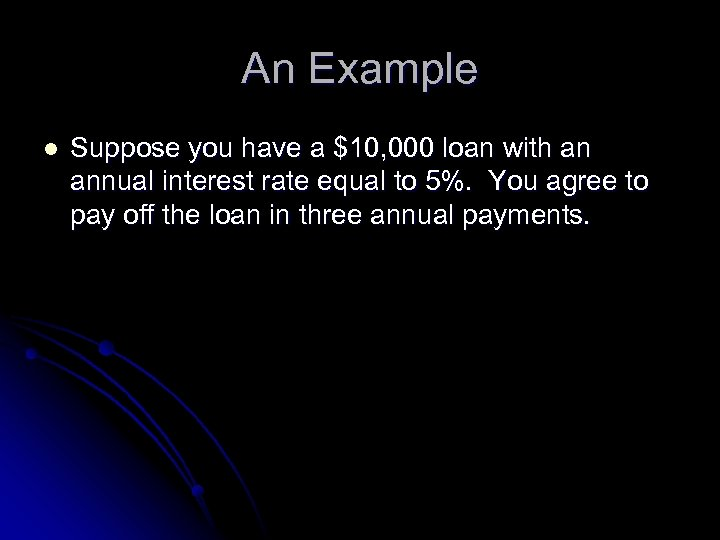An Example l Suppose you have a \$10, 000 loan with an annual interest rate equal to 5%. You agree to pay off the loan in three annual payments.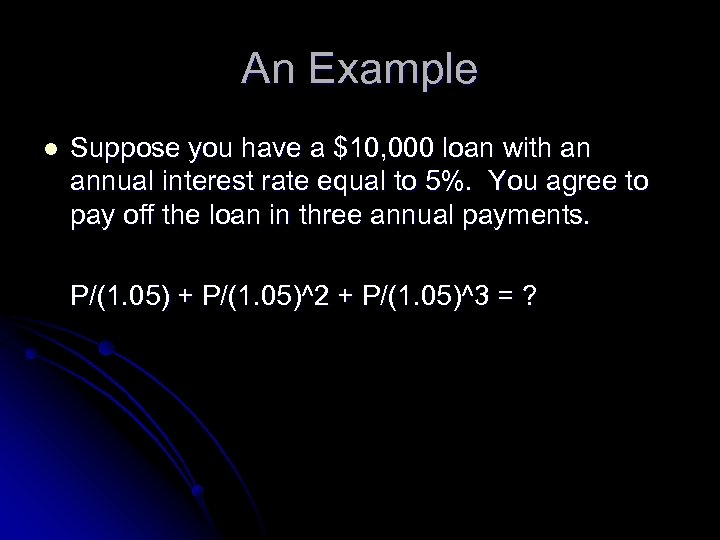An Example l Suppose you have a \$10, 000 loan with an annual interest rate equal to 5%. You agree to pay off the loan in three annual payments. P/(1. 05) + P/(1. 05)^2 + P/(1. 05)^3 = ?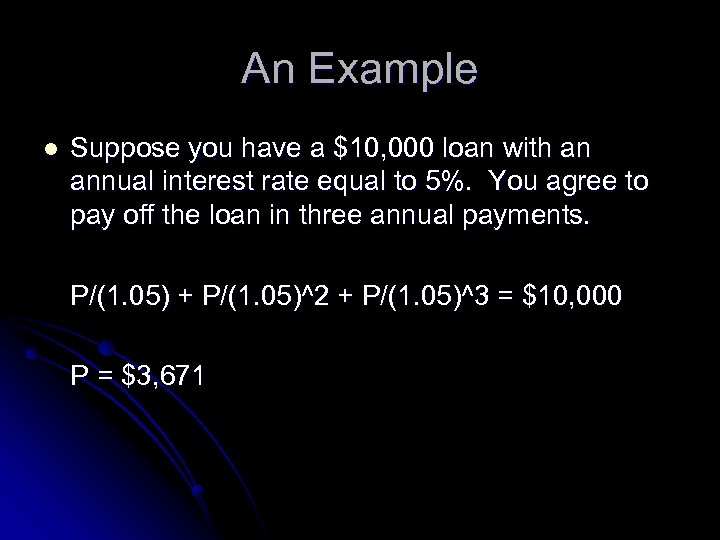An Example l Suppose you have a \$10, 000 loan with an annual interest rate equal to 5%. You agree to pay off the loan in three annual payments. P/(1. 05) + P/(1. 05)^2 + P/(1. 05)^3 = \$10, 000 P = \$3, 671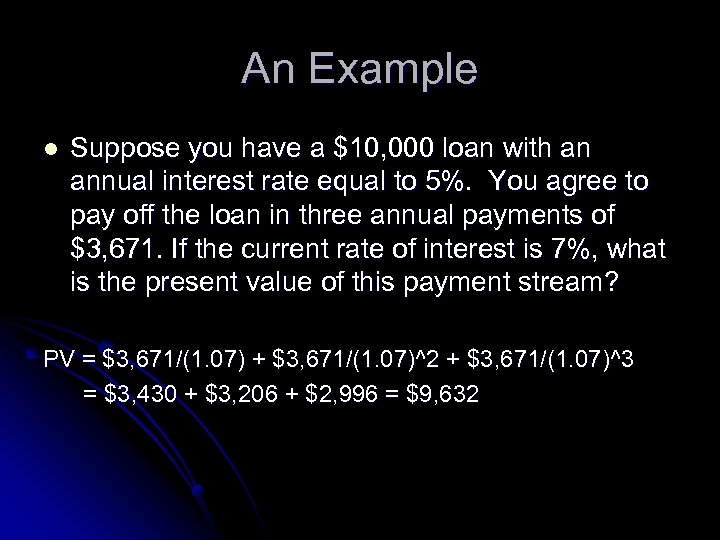An Example l Suppose you have a \$10, 000 loan with an annual interest rate equal to 5%. You agree to pay off the loan in three annual payments of \$3, 671. If the current rate of interest is 7%, what is the present value of this payment stream? PV = \$3, 671/(1. 07) + \$3, 671/(1. 07)^2 + \$3, 671/(1. 07)^3 = \$3, 430 + \$3, 206 + \$2, 996 = \$9, 632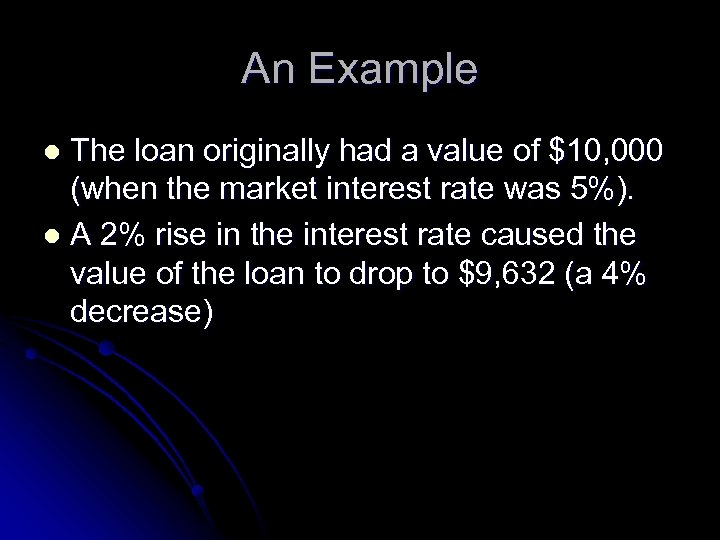An Example The loan originally had a value of \$10, 000 (when the market interest rate was 5%). l A 2% rise in the interest rate caused the value of the loan to drop to \$9, 632 (a 4% decrease) l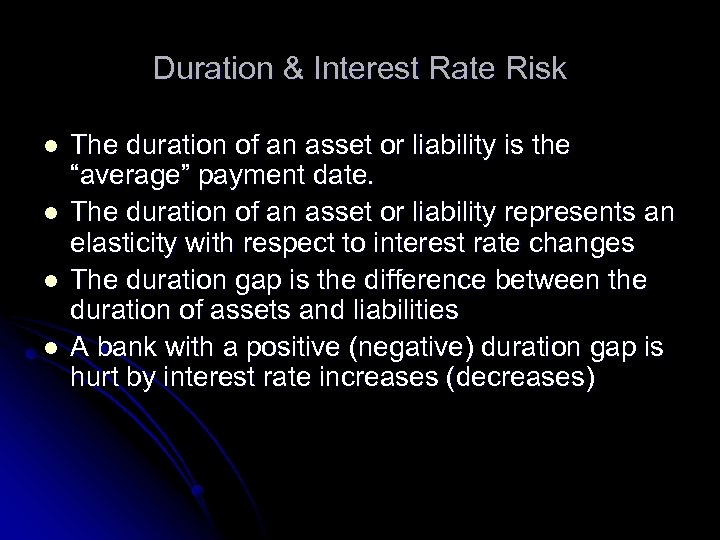Duration & Interest Rate Risk l l The duration of an asset or liability is the “average” payment date. The duration of an asset or liability represents an elasticity with respect to interest rate changes The duration gap is the difference between the duration of assets and liabilities A bank with a positive (negative) duration gap is hurt by interest rate increases (decreases)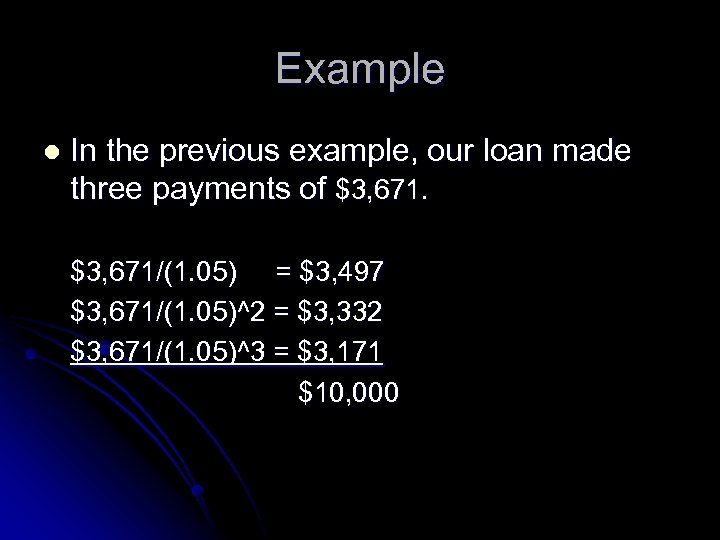Example l In the previous example, our loan made three payments of \$3, 671/(1. 05) = \$3, 497 \$3, 671/(1. 05)^2 = \$3, 332 \$3, 671/(1. 05)^3 = \$3, 171 \$10, 000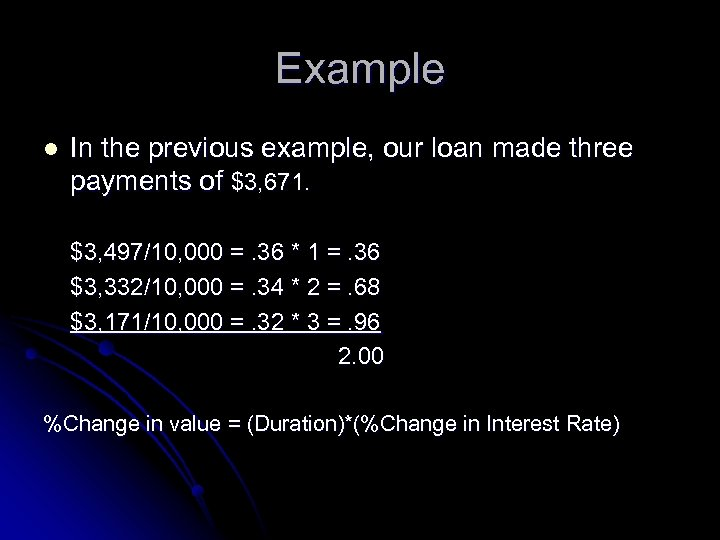Example l In the previous example, our loan made three payments of \$3, 671. \$3, 497/10, 000 =. 36 * 1 =. 36 \$3, 332/10, 000 =. 34 * 2 =. 68 \$3, 171/10, 000 =. 32 * 3 =. 96 2. 00 %Change in value = (Duration)*(%Change in Interest Rate)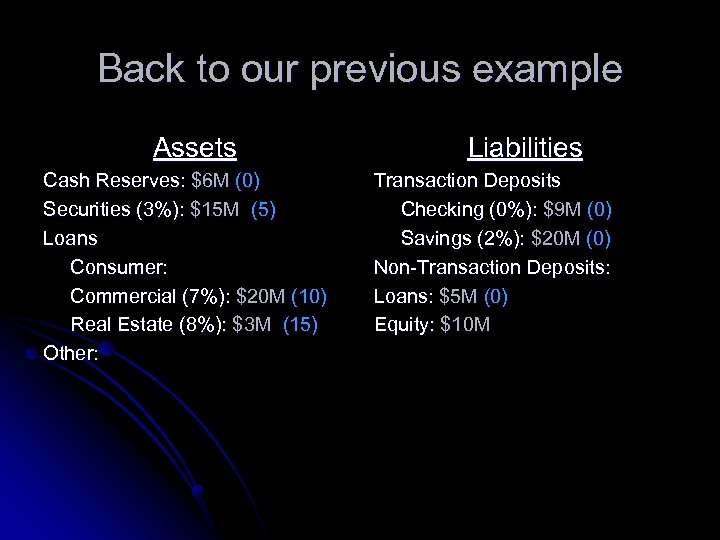Back to our previous example Assets Cash Reserves: \$6 M (0) Securities (3%): \$15 M (5) Loans Consumer: Commercial (7%): \$20 M (10) Real Estate (8%): \$3 M (15) Other: Liabilities Transaction Deposits Checking (0%): \$9 M (0) Savings (2%): \$20 M (0) Non-Transaction Deposits: Loans: \$5 M (0) Equity: \$10 M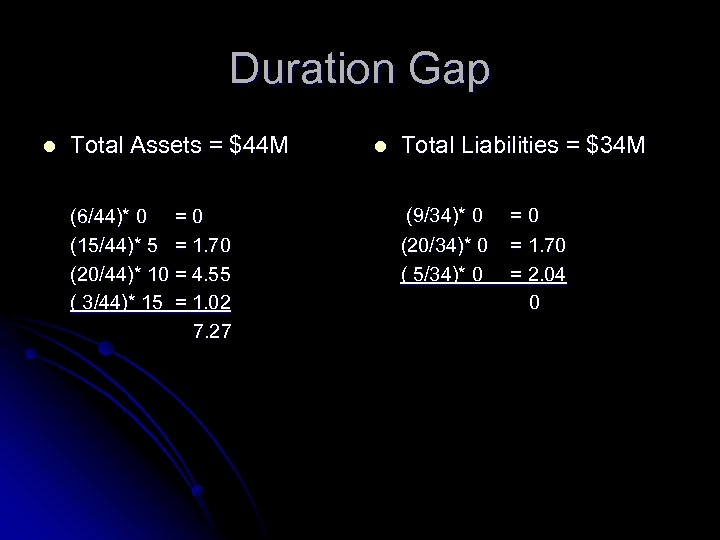Duration Gap l Total Assets = \$44 M (6/44)* 0 = 0 (15/44)* 5 = 1. 70 (20/44)* 10 = 4. 55 ( 3/44)* 15 = 1. 02 7. 27 l Total Liabilities = \$34 M (9/34)* 0 (20/34)* 0 ( 5/34)* 0 =0 = 1. 70 = 2. 04 0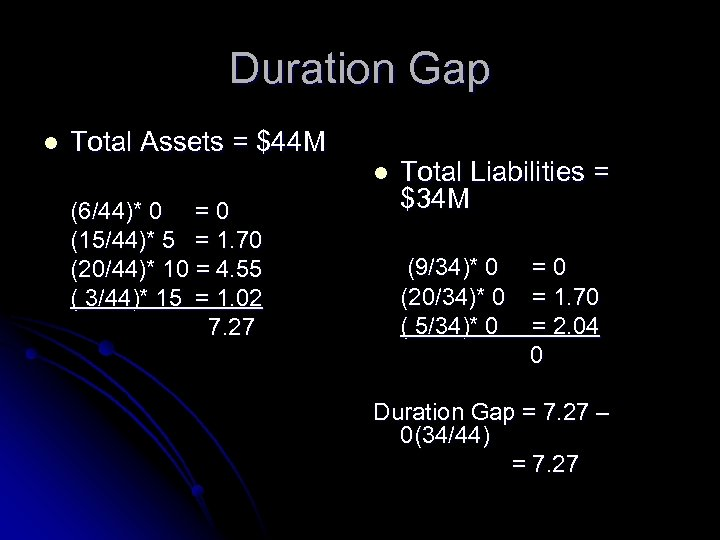Duration Gap l Total Assets = \$44 M l (6/44)* 0 = 0 (15/44)* 5 = 1. 70 (20/44)* 10 = 4. 55 ( 3/44)* 15 = 1. 02 7. 27 Total Liabilities = \$34 M (9/34)* 0 (20/34)* 0 ( 5/34)* 0 =0 = 1. 70 = 2. 04 0 Duration Gap = 7. 27 – 0(34/44) = 7. 27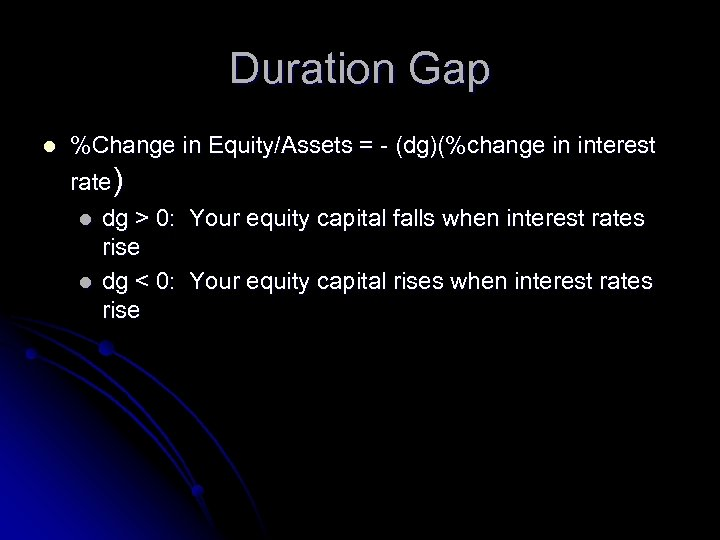Duration Gap l %Change in Equity/Assets = - (dg)(%change in interest rate) l l dg > 0: Your equity capital falls when interest rates rise dg < 0: Your equity capital rises when interest rates rise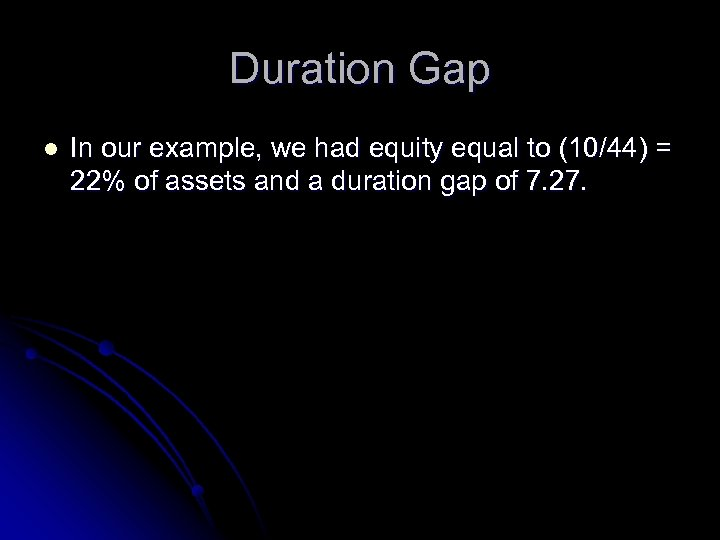Duration Gap l In our example, we had equity equal to (10/44) = 22% of assets and a duration gap of 7. 27.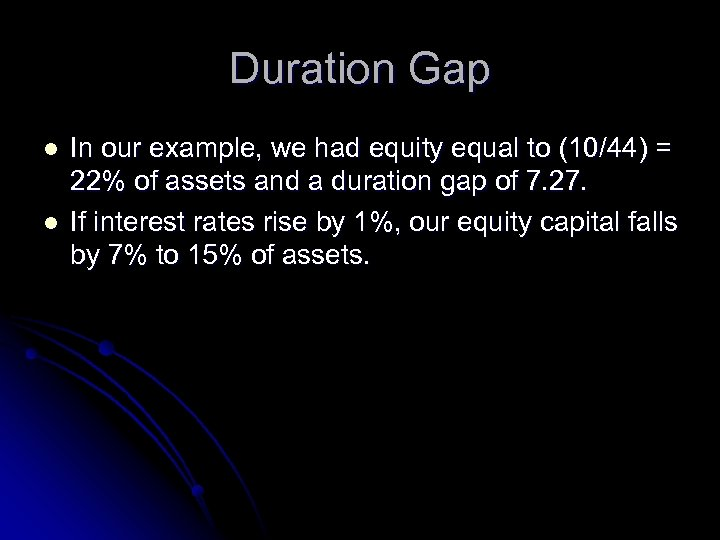Duration Gap l l In our example, we had equity equal to (10/44) = 22% of assets and a duration gap of 7. 27. If interest rates rise by 1%, our equity capital falls by 7% to 15% of assets.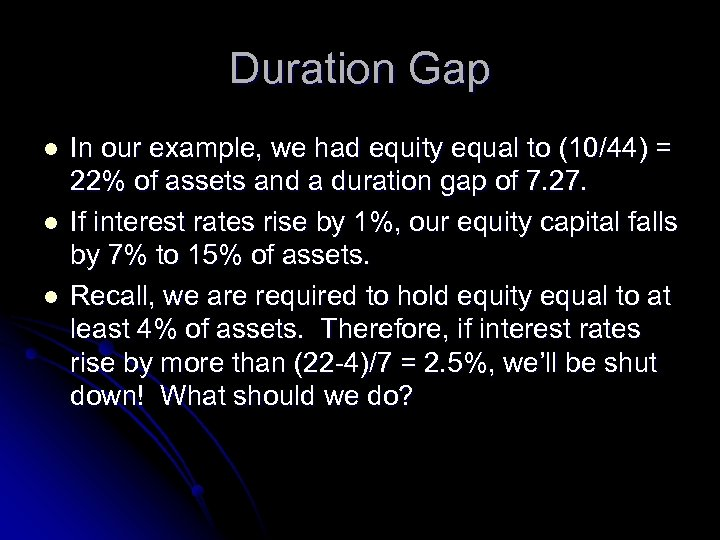Duration Gap l l l In our example, we had equity equal to (10/44) = 22% of assets and a duration gap of 7. 27. If interest rates rise by 1%, our equity capital falls by 7% to 15% of assets. Recall, we are required to hold equity equal to at least 4% of assets. Therefore, if interest rates rise by more than (22 -4)/7 = 2. 5%, we’ll be shut down! What should we do?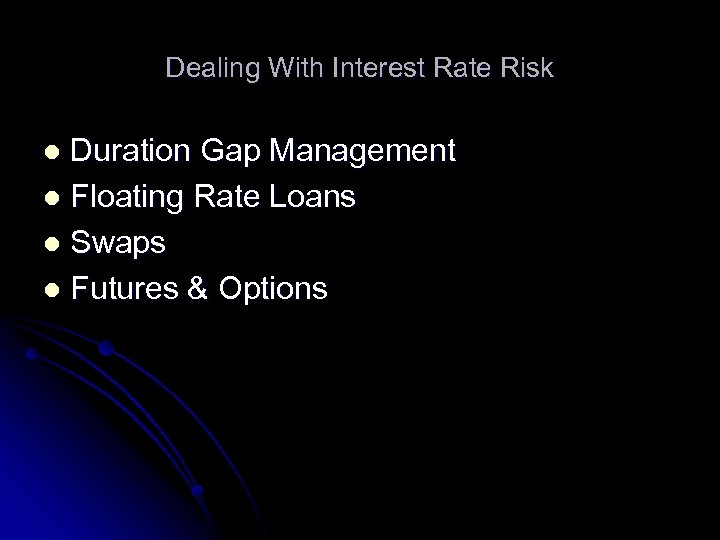Dealing With Interest Rate Risk Duration Gap Management l Floating Rate Loans l Swaps l Futures & Options l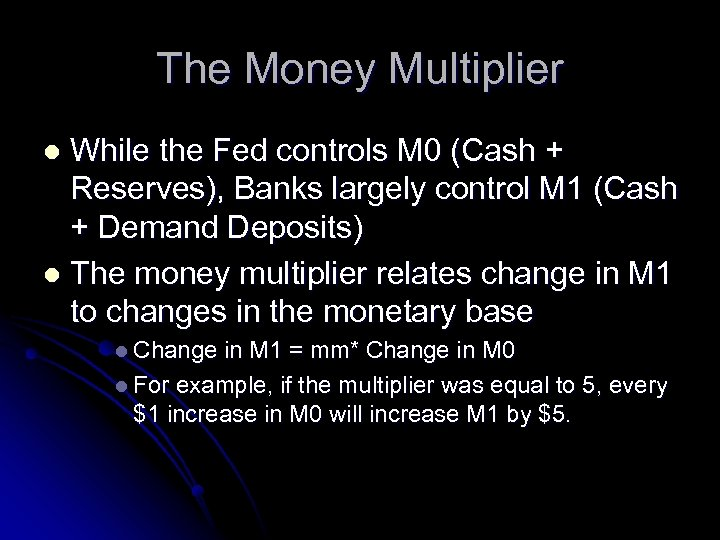The Money Multiplier While the Fed controls M 0 (Cash + Reserves), Banks largely control M 1 (Cash + Demand Deposits) l The money multiplier relates change in M 1 to changes in the monetary base l l Change in M 1 = mm* Change in M 0 l For example, if the multiplier was equal to 5, every \$1 increase in M 0 will increase M 1 by \$5.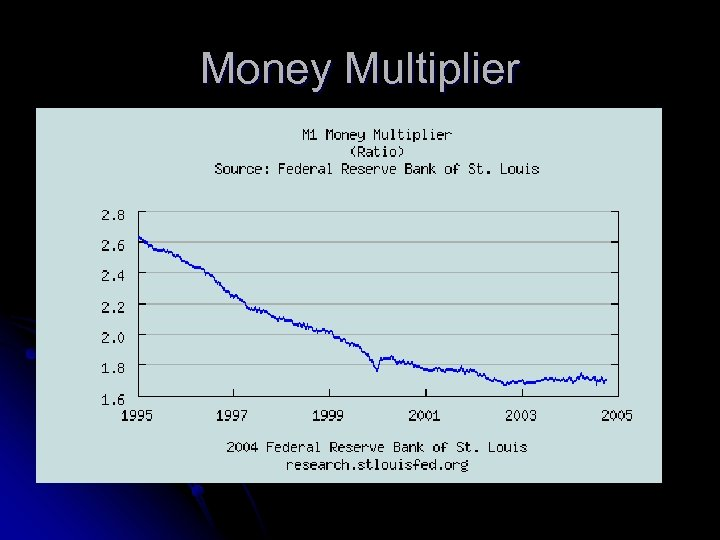Money Multiplier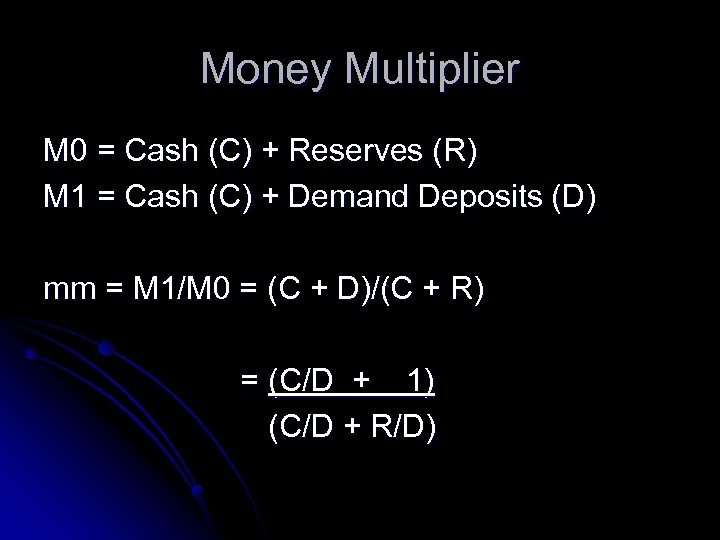Money Multiplier M 0 = Cash (C) + Reserves (R) M 1 = Cash (C) + Demand Deposits (D) mm = M 1/M 0 = (C + D)/(C + R) = (C/D + 1) (C/D + R/D)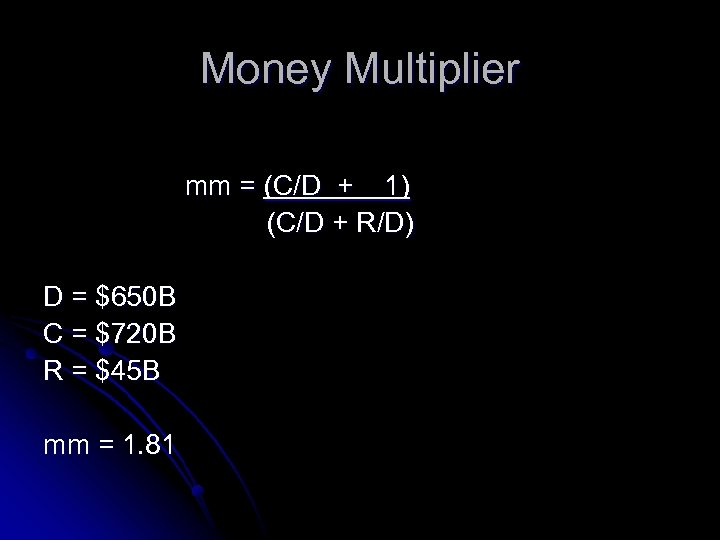Money Multiplier mm = (C/D + 1) (C/D + R/D) D = \$650 B C = \$720 B R = \$45 B mm = 1. 81